Q-Chem 5.1 User’s Manual

# 7.3 Time-Dependent Density Functional Theory (TDDFT)

## 7.3.1 Brief Introduction to TDDFT

Excited states may be obtained from density functional theory by time-dependent density functional theory, which calculates poles in the response of the ground state density to a time-varying applied electric field. These poles are Bohr frequencies, or in other words the excitation energies. Operationally, this involves solution of an eigenvalue equation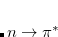(7.15)

where the elements of the matrixsimilar to those used at the CIS level, Eq. eq:orbital-Hessian, but with an exchange-correlation correction. Elements of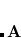are similar. Equation eq:TDDFT is solved iteratively for the lowest few excitation energies,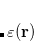. Alternatively, one can make a CIS-like Tamm-Dancoff approximation (TDA), in which the “de-excitation” amplitudes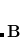are neglected, thematrix is not required, and Eq. eq:TDDFT reduces to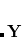.

TDDFT is popular because its computational cost is roughly similar to that of the simple CIS method, but a description of differential electron correlation effects is implicit in the method. It is advisable to only employ TDDFT for low-lying valence excited states that are below the first ionization potential of the molecule, or more conservatively, below the first Rydberg state, and in such cases the valence excitation energies are often remarkably improved relative to CIS, with an accuracy of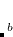0.3 eV for many functionals. The calculation of the nuclear gradients of full TDDFT and within the TDA is implemented.

On the other hand, standard density functionals do not yield a potential with the correct long-range Coulomb tail, owing to the so-called self-interaction problem, and therefore excitation energies corresponding to states that sample this tail (e.g., diffuse Rydberg states and some charge transfer excited states) are not given accurately. The extent to which a particular excited state is characterized by charge transfer can be assessed using an a spatial overlap metric proposed by Peach, Benfield, Helgaker, and Tozer (PBHT). (However, see Ref. Richard:2011 for a cautionary note regarding this metric.)

Standard TDDFT also does not yield a good description of static correlation effects (see Section 6.10), because it is based on a single reference configuration of Kohn-Sham orbitals. Recently, a new variation of TDDFT called spin-flip (SF) DFT was developed by Yihan Shao, Martin Head-Gordon and Anna Krylov to address this issue. SF-DFT is different from standard TDDFT in two ways:

• The reference is a high-spin triplet (quartet) for a system with an even (odd) number of electrons;

• One electron is spin-flipped from an alpha Kohn-Sham orbital to a beta orbital during the excitation.

SF-DFT can describe the ground state as well as a few low-lying excited states, and has been applied to bond-breaking processes, and di- and tri-radicals with degenerate or near-degenerate frontier orbitals. Recently, we also implemented a SF-DFT method with a non-collinear exchange-correlation potential from Tom Ziegler et al., which is in many case an improvement over collinear SF-DFT. Recommended functionals for SF-DFT calculations are 5050 and PBE50 (see Ref. Shao:2012 for extensive benchmarks). See also Section 7.7.3 for details on wave function-based spin-flip models.

## 7.3.2 TDDFT within a Reduced Single-Excitation Space

Much of chemistry and biology occurs in solution or on surfaces. The molecular environment can have a large effect on electronic structure and may change chemical behavior. Q-Chem is able to compute excited states within a local region of a system through performing the TDDFT (or CIS) calculation with a reduced single excitation subspace, in which some of the amplitudes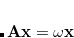in Eq. eq:TDDFT are excluded. (This is implemented within the TDA, so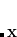.) This allows the excited states of a solute molecule to be studied with a large number of solvent molecules reducing the rapid rise in computational cost. The success of this approach relies on there being only weak mixing between the electronic excitations of interest and those omitted from the single excitation space. For systems in which there are strong hydrogen bonds between solute and solvent, it is advisable to include excitations associated with the neighboring solvent molecule(s) within the reduced excitation space.

The reduced single excitation space is constructed from excitations between a subset of occupied and virtual orbitals. These can be selected from an analysis based on Mulliken populations and molecular orbital coefficients. For this approach the atoms that constitute the solvent needs to be defined. Alternatively, the orbitals can be defined directly. The atoms or orbitals are specified within a $solute block. These approach is implemented within the TDA and has been used to study the excited states of formamide in solution, CO on the Pt(111) surface, and the tryptophan chromophore within proteins. ## 7.3.3 Job Control for TDDFT Input for time-dependent density functional theory calculations follows very closely the input already described for the uncorrelated excited state methods described in the previous section (in particular, see Section 7.2.8). There are several points to be aware of: • The exchange and correlation functionals are specified exactly as for a ground state DFT calculation, through EXCHANGE and CORRELATION. • If RPA is set to TRUE, a “full” TDDFT calculation will be performed, however the default value is RPA = FALSE, which invokes the TDA, in which the de-excitation amplitudesin Eq. eq:TDDFT are neglected, which is usually a good approximation for excitation energies, although oscillator strengths within the TDA no longer formally satisfy the Thomas-Reiche-Kuhn sum rule. For RPA = TRUE, a TDA calculation is performed first and used as the initial guess for the full TDDFT calculation. The TDA calculation can be skipped altogether using RPA = 2 • If SPIN_FLIP is set to TRUE when performing a TDDFT calculation, a SF-DFT calculation will also be performed. At present, SF-DFT is only implemented within TDDFT/TDA so RPA must be set to FALSE. Remember to set the spin multiplicity to 3 for systems with an even-number of electrons (e.g., diradicals), and 4 for odd-number electron systems (e.g., triradicals). TRNSS  Controls whether reduced single excitation space is used. TYPE:  LOGICAL DEFAULT:  FALSE Use full excitation space. OPTIONS:  TRUE Use reduced excitation space. RECOMMENDATION:  None TRTYPE  Controls how reduced subspace is specified. TYPE:  INTEGER DEFAULT:  1 OPTIONS:  1 Select orbitals localized on a set of atoms. 2 Specify a set of orbitals. 3 Specify a set of occupied orbitals, include excitations to all virtual orbitals. RECOMMENDATION:  None N_SOL  Specifies number of atoms or orbitals in the$solute section.

TYPE:
 INTEGER

DEFAULT:
 No default.

OPTIONS:
 User defined.

RECOMMENDATION:
 None

CISTR_PRINT
 Controls level of output.

TYPE:
 LOGICAL

DEFAULT:
 FALSE Minimal output.

OPTIONS:
 TRUE Increase output level.

RECOMMENDATION:
 None

CUTOCC
 Specifies occupied orbital cutoff.

TYPE:
 INTEGER

DEFAULT:
 50

OPTIONS:
 0-200 CUTOFF = CUTOCC/100

RECOMMENDATION:
 None

CUTVIR
 Specifies virtual orbital cutoff.

TYPE:
 INTEGER

DEFAULT:
 0 No truncation

OPTIONS:
 0-100 CUTOFF = CUTVIR/100

RECOMMENDATION:
 None

PBHT_ANALYSIS
 Controls whether overlap analysis of electronic excitations is performed.

TYPE:
 LOGICAL

DEFAULT:
 FALSE

OPTIONS:
 FALSE Do not perform overlap analysis. TRUE Perform overlap analysis.

RECOMMENDATION:
 None

PBHT_FINE
 Increases accuracy of overlap analysis.

TYPE:
 LOGICAL

DEFAULT:
 FALSE

OPTIONS:
 FALSE TRUE Increase accuracy of overlap analysis.

RECOMMENDATION:
 None

SRC_DFT
 Selects form of the short-range corrected functional.

TYPE:
 INTEGER

DEFAULT:
 No default

OPTIONS:
 1 SRC1 functional. 2 SRC2 functional.

RECOMMENDATION:
 None

OMEGA
 Sets the Coulomb attenuation parameter for the short-range component.

TYPE:
 INTEGER

DEFAULT:
 No default

OPTIONS: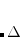Corresponding to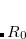, in units of bohr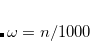RECOMMENDATION:
 None

OMEGA2
 Sets the Coulomb attenuation parameter for the long-range component.

TYPE:
 INTEGER

DEFAULT:
 No default

OPTIONS:Corresponding to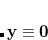, in units of bohrRECOMMENDATION:
 None

HF_SR
 Sets the fraction of Hartree-Fock exchange at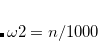.

TYPE:
 INTEGER

DEFAULT:
 No default

OPTIONS:Corresponding to HF_SR =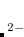RECOMMENDATION:
 None

HF_LR
 Sets the fraction of Hartree-Fock exchange at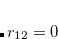.

TYPE:
 INTEGER

DEFAULT:
 No default

OPTIONS:Corresponding to HF_LR =RECOMMENDATION:
 None

WANG_ZIEGLER_KERNEL
 Controls whether to use the Wang-Ziegler non-collinear exchange-correlation kernel in a SF-DFT calculation.

TYPE:
 LOGICAL

DEFAULT:
 FALSE

OPTIONS:
 FALSE Do not use non-collinear kernel. TRUE Use non-collinear kernel.

RECOMMENDATION:
 None

## 7.3.4 TDDFT Coupled with C-PCM for Excitation Energies and Properties Calculations

As described in Section 12.2 (and especially Section 12.2.2), continuum solvent models such as C-PCM allow one to include solvent effect in the calculations. TDDFT/C-PCM allows excited-state modeling in solution. Q-Chem also features TDDFT coupled with C-PCM which extends TDDFT to calculations of properties of electronically-excited molecules in solution. In particular, TDDFT/C-PCM allows one to perform geometry optimization and vibrational analysis.

When TDDFT/C-PCM is applied to calculate vertical excitation energies, the solvent around vertically excited solute is out of equilibrium. While the solvent electron density equilibrates fast to the density of the solute (electronic response), the relaxation of nuclear degrees of freedom (e.g., orientational polarization) takes place on a slower timescale. To describe this situation, an optical dielectric constant is employed. To distinguish between equilibrium and non-equilibrium calculations, two dielectric constants are used in these calculations: a static constant (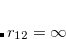), equal to the equilibrium bulk value, and a fast constant (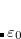) related to the response of the medium to high frequency perturbations. For vertical excitation energy calculations (corresponding to the unrelaxed solvent nuclear degrees of freedom), it is recommended to use the optical dielectric constant for), whereas for the geometry optimization and vibrational frequency calculations, the static dielectric constant should be used.

The example below illustrates TDDFT/C-PCM calculations of vertical excitation energies. More information concerning the C-PCM and the various PCM job control options can be found in Section 12.2.

Example 7.105  TDDFT/C-PCM low-lying vertical excitation energy

$molecule 0 1 C 0.0 0.0 0.0 O 0.0 0.0 1.21$end

$rem EXCHANGE B3lyp CIS_N_ROOTS 10 CIS_SINGLETS true CIS_TRIPLETS true RPA TRUE BASIS 6-31+G* XC_GRID 1 SOLVENT_METHOD pcm$end

$pcm Theory CPCM Method SWIG Solver Inversion Radii Bondi$end

$solvent Dielectric 78.39 OpticalDielectric 1.777849$end


## 7.3.5 Analytical Excited-State Hessian in TDDFT

To carry out vibrational frequency analysis of an excited state with TDDFT, an optimization of the excited-state geometry is always necessary. Like the vibrational frequency analysis of the ground state, the frequency analysis of the excited state should be also performed at a stationary point on the excited state potential surface. The $rem variable CIS_STATE_DERIV should be set to the excited state for which an optimization and frequency analysis is needed, in addition to the$rem keywords used for an excitation energy calculation.

Compared to the numerical differentiation method, the analytical calculation of geometrical second derivatives of the excitation energy needs much less time but much more memory. The computational cost is mainly consumed by the steps to solve both the CPSCF equations for the derivatives of molecular orbital coefficients C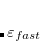and the CP-TDDFT equations for the derivatives of the transition vectors, as well as to build the Hessian matrix. The memory usages for these steps scale as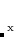, where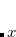is the number of basis functions and m is the number of atoms. For large systems, it is thus essential to solve all the coupled-perturbed equations in segments. In this case, the $rem variable CPSCF_NSEG is always needed. In the calculation of the analytical TDDFT excited-state Hessian, one has to evaluate a large number of energy-functional derivatives: the first-order to fourth-order functional derivatives with respect to the density variables as well as their derivatives with respect to the nuclear coordinates. Therefore, a very fine integration grid for DFT calculation should be adapted to guarantee the accuracy of the results. Analytical TDDFT/C-PCM Hessian has been implemented in Q-Chem. Normal mode analysis for a system in solution can be performed with the frequency calculation by TDDFT/C-PCM method. The$rem and $pcm variables for the excited state calculation with TDDFT/C-PCM included in the vertical excitation energy example above are needed. When the properties of large systems are calculated, you must pay attention to the memory limit. At present, only a few exchange correlation functionals, including Slater+VWN, BLYP, B3LYP, are available for the analytical Hessian calculation. Example 7.106 A B3LYP/6-31G* optimization in gas phase, followed by a frequency analysis for the first excited state of the peroxy radical $molecule
0 2
C  1.004123  -0.180454   0.000000
O -0.246002   0.596152   0.000000
O -1.312366  -0.230256   0.000000
H  1.810765   0.567203   0.000000
H  1.036648  -0.805445  -0.904798
H  1.036648  -0.805445   0.904798
$end$rem
JOBTYPE           opt
EXCHANGE          b3lyp
CIS_STATE_DERIV   1
BASIS             6-31G*
CIS_N_ROOTS       10
CIS_SINGLETS      true
CIS_TRIPLETS      false
XC_GRID           000075000302
RPA               0
$end @@@$molecule
$end$rem
JOBTYPE           freq
EXCHANGE          b3lyp
CIS_STATE_DERIV   1
BASIS             6-31G*
CIS_N_ROOTS       10
CIS_SINGLETS      true
CIS_TRIPLETS      false
XC_GRID           000075000302
RPA               0
$end  Example 7.107 The optimization and Hessian calculation for low-lying excited state of 9-Fluorenone + 2 methanol in methanol solution using TDDFT/C-PCM $molecule
0 1
6   -1.987249    0.699711    0.080583
6   -1.987187   -0.699537   -0.080519
6   -0.598049   -1.148932   -0.131299
6    0.282546    0.000160    0.000137
6   -0.598139    1.149219    0.131479
6   -0.319285   -2.505397   -0.285378
6   -1.386049   -3.395376   -0.388447
6   -2.743097   -2.962480   -0.339290
6   -3.049918   -1.628487   -0.186285
6   -3.050098    1.628566    0.186246
6   -2.743409    2.962563    0.339341
6   -1.386397    3.395575    0.388596
6   -0.319531    2.505713    0.285633
8    1.560568    0.000159    0.000209
1    0.703016   -2.862338   -0.324093
1   -1.184909   -4.453877   -0.510447
1   -3.533126   -3.698795   -0.423022
1   -4.079363   -1.292006   -0.147755
1    0.702729    2.862769    0.324437
1   -1.185378    4.454097    0.510608
1   -3.533492    3.698831    0.422983
1   -4.079503    1.291985    0.147594
8    3.323150    2.119222    0.125454
1    2.669309    1.389642    0.084386
6    3.666902    2.489396   -1.208239
1    4.397551    3.298444   -1.151310
1    4.116282    1.654650   -1.759486
1    2.795088    2.849337   -1.768206
1    2.669205   -1.389382   -0.084343
8    3.322989   -2.119006   -0.125620
6    3.666412   -2.489898    1.207974
1    4.396966   -3.299023    1.150789
1    4.115800   -1.655485    1.759730
1    2.794432   -2.850001    1.767593
$end$rem
JOBTYPE             OPT
EXCHANGE            B3lyp
CIS_N_ROOTS         10
CIS_SINGLETS        true
CIS_TRIPLETS        true
CIS_STATE_DERIV     1   Lowest TDDFT state
RPA                 TRUE
BASIS               6-311G**
XC_GRID             000075000302
SOLVENT_METHOD      pcm
$end$pcm
Theory      CPCM
Method      SWIG
Solver      Inversion
$end$solvent
Dielectric 32.613
$end @@@$molecule
$end$rem
JOBTYPE           freq
EXCHANGE          B3lyp
CIS_N_ROOTS       10
CIS_SINGLETS      true
CIS_TRIPLETS      true
RPA               TRUE
CIS_STATE_DERIV   1   Lowest TDDFT state
BASIS             6-311G**
XC_GRID           000075000302
SOLVENT_METHOD    pcm
MEM_STATIC        4000
MEM_TOTAL         24000
CPSCF_NSEG        3
$end$pcm
Theory      CPCM
Method      SWIG
Solver      Inversion
$end$solvent
Dielectric  32.613
$end  ## 7.3.6 Calculations of Spin-Orbit Couplings Between TDDFT States Calculations of spin-orbit couplings (SOCs) for TDDFT states within the Tamm-Dancoff approximation or RPA (including TDHF and CIS states) is available. We employ the one-electron Breit Pauli Hamiltonian to calculate the SOC constant between TDDFT states.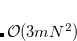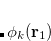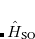(7.16) where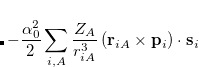denotes electrons,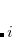denotes nuclei,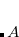is the fine structure constant. Z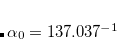is the bare positive charge on nucleus A. In the second quantization representation, the spin-orbit Hamiltonian in different directions can be expressed as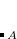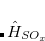(7.17)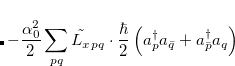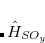(7.18)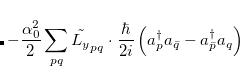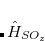(7.19) where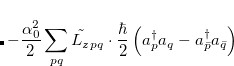. The single-reference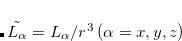excited states (within the Tamm-Dancoff approximation) are given by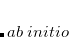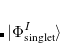(7.20)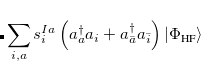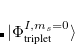(7.21)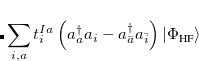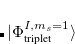(7.22)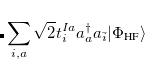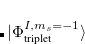(7.23) where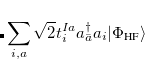and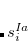are singlet and triplet excitation coefficients of the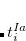singlet or triplet state respectively, with the normalization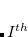;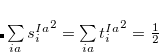refers to the Hartree-Fock ground state. Thus the SOC constant from the singlet state to different triplet manifolds can be obtained as follows,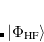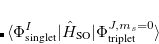(7.24)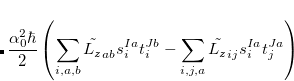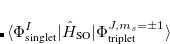(7.25)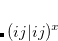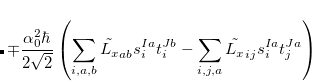(7.26) The SOC constant between different triplet manifolds can be obtained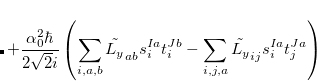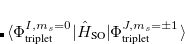(7.27)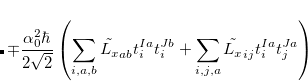(7.28)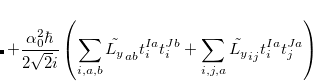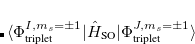(7.29) Note that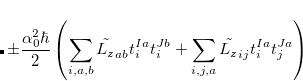. The total (root-mean-square) spin-orbit coupling is given by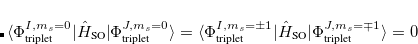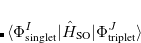(7.30)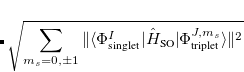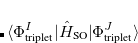(7.31) For RPA states, the SOC constant can simply be obtained by replacing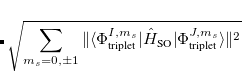(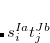) with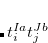(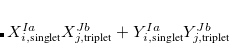) Setting the$rem variable CALC_SOC = TRUE will enable the SOC calculation for all calculated TDDFT states.

CALC_SOC
 Controls whether to calculate the SOC constants for EOM-CC, ADC, TDDFT/TDA and TDDFT.

TYPE:
 LOGICAL

DEFAULT:
 FALSE

OPTIONS:
 FALSE Do not perform the SOC calculation. TRUE Perform the SOC calculation.

RECOMMENDATION:
 None

Example 7.108  Calculation of SOCs for water molecule using TDDFT/B3LYP functional within the TDA.

$comment This sample input calculates the spin-orbit coupling constants for water between its ground state and its TDDFT/TDA excited triplets as well as the coupling between its TDDFT/TDA singlets and triplets. Results are given in cm-1.$end

$molecule 0 1 H 0.000000 -0.115747 1.133769 H 0.000000 1.109931 -0.113383 O 0.000000 0.005817 -0.020386$end

$rem JOBTYPE sp EXCHANGE b3lyp BASIS 6-31G CIS_N_ROOTS 4 CIS_CONVERGENCE 8 CORRELATION none MAX_SCF_CYCLES 600 MAX_CIS_CYCLES 50 SCF_ALGORITHM diis MEM_STATIC 300 MEM_TOTAL 2000 SYMMETRY false SYM_IGNORE true UNRESTRICTED false CIS_SINGLETS true CIS_TRIPLETS true CALC_SOC true SET_ITER 300$end


## 7.3.7 Various TDDFT-Based Examples

Example 7.109  This example shows two jobs which request variants of time-dependent density functional theory calculations. The first job, using the default value of RPA = FALSE, performs TDDFT in the Tamm-Dancoff approximation (TDA). The second job, with RPA = TRUE performs a both TDA and full TDDFT calculations.

$comment methyl peroxy radical TDDFT/TDA and full TDDFT with 6-31+G*$end

$molecule 0 2 C 1.00412 -0.18045 0.00000 O -0.24600 0.59615 0.00000 O -1.31237 -0.23026 0.00000 H 1.81077 0.56720 0.00000 H 1.03665 -0.80545 -0.90480 H 1.03665 -0.80545 0.90480$end

$rem EXCHANGE b CORRELATION lyp CIS_N_ROOTS 5 BASIS 6-31+G* SCF_CONVERGENCE 7$end

@@@

$molecule read$end

$rem EXCHANGE b CORRELATION lyp CIS_N_ROOTS 5 RPA true BASIS 6-31+G* SCF_CONVERGENCE 7$end


Example 7.110  This example shows a calculation of the excited states of a formamide-water complex within a reduced excitation space of the orbitals located on formamide

$comment formamide-water TDDFT/TDA in reduced excitation space$end

$molecule 0 1 H 1.13 0.49 -0.75 C 0.31 0.50 -0.03 N -0.28 -0.71 0.08 H -1.09 -0.75 0.67 H 0.23 -1.62 -0.22 O -0.21 1.51 0.47 O -2.69 1.94 -0.59 H -2.59 2.08 -1.53 H -1.83 1.63 -0.30$end

$rem EXCHANGE b3lyp CIS_N_ROOTS 10 BASIS 6-31++G** TRNSS TRUE TRTYPE 1 CUTOCC 60 CUTVIR 40 CISTR_PRINT TRUE$end

$solute 1 2 3 4 5 6$end


Example 7.111  This example shows a calculation of the core-excited states at the oxygen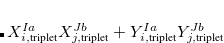-edge of CO with a short-range corrected functional.

$comment TDDFT with short-range corrected (SRC1) functional for the oxygen K-edge of CO$end

$molecule 0 1 C 0.000000 0.000000 -0.648906 O 0.000000 0.000000 0.486357$end

$rem EXCHANGE SRC1-R1 BASIS 6-311(2+,2+)G** CIS_N_ROOTS 6 CIS_TRIPLETS false TRNSS true TRTYPE 3 N_SOL 1$end

$solute 1$end


Example 7.112  This example shows a calculation of the core-excited states at the phosphorus-edge with a short-range corrected functional.

$comment TDDFT with short-range corrected (SRC2) functional for the phosphorus K-edge of PH3$end

$molecule 0 1 H 1.196206 0.000000 -0.469131 P 0.000000 0.000000 0.303157 H -0.598103 -1.035945 -0.469131 H -0.598103 1.035945 -0.469131$end

$rem EXCHANGE SRC2-R2 BASIS 6-311(2+,2+)G** CIS_N_ROOTS 6 CIS_TRIPLETS false TRNSS true TRTYPE 3 N_SOL 1$end

$solute 1$end


Example 7.113  SF-TDDFT SP calculation of the 6 lowest states of the TMM diradical using recommended 50-50 functional

$molecule 0 3 C C 1 CC1 C 1 CC2 2 A2 C 1 CC2 2 A2 3 180.0 H 2 C2H 1 C2CH 3 0.0 H 2 C2H 1 C2CH 4 0.0 H 3 C3Hu 1 C3CHu 2 0.0 H 3 C3Hd 1 C3CHd 4 0.0 H 4 C3Hu 1 C3CHu 2 0.0 H 4 C3Hd 1 C3CHd 3 0.0 CC1 = 1.35 CC2 = 1.47 C2H = 1.083 C3Hu = 1.08 C3Hd = 1.08 C2CH = 121.2 C3CHu = 120.3 C3CHd = 121.3 A2 = 121.0$end

$rem EXCHANGE gen BASIS 6-31G* SCF_GUESS core SCF_CONVERGENCE 10 MAX_SCF_CYCLES 100 SPIN_FLIP 1 CIS_N_ROOTS 6 CIS_CONVERGENCE 10 MAX_CIS_CYCLES 100$end

$xc_functional X HF 0.50 X S 0.08 X B 0.42 C VWN 0.19 C LYP 0.81$end


Example 7.114  SF-DFT with non-collinear exchange-correlation functional for low-lying states of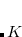$comment Non-collinear SF-DFT calculation for CH2 at 3B1 state geometry from EOM-CCSD(fT) calculation$end

$molecule 0 3 C H 1 rCH H 1 rCH 2 HCH rCH = 1.0775 HCH = 133.29$end

$rem EXCHANGE PBE0 BASIS cc-pVTZ SPIN_FLIP 1 WANG_ZIEGLER_KERNEL TRUE SCF_CONVERGENCE 10 CIS_N_ROOTS 6 CIS_CONVERGENCE 10$end